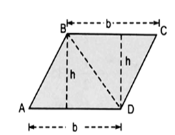# 1. We know that parallelogram is also a quadrilateral. Let us also split such a quadrilateral into two triangles, find their areas  and hence that of the parallelogram. Does this agree with the formula that you know already? (Fig 11.12)S seema garhwalThis agree with the formula that we know already.

Exams
Articles
Questions